# 2.3: Understanding Graphs of Functions

$$\newcommand{\vecs}{\overset { \rightharpoonup} {\mathbf{#1}} }$$ $$\newcommand{\vecd}{\overset{-\!-\!\rightharpoonup}{\vphantom{a}\smash {#1}}}$$$$\newcommand{\id}{\mathrm{id}}$$ $$\newcommand{\Span}{\mathrm{span}}$$ $$\newcommand{\kernel}{\mathrm{null}\,}$$ $$\newcommand{\range}{\mathrm{range}\,}$$ $$\newcommand{\RealPart}{\mathrm{Re}}$$ $$\newcommand{\ImaginaryPart}{\mathrm{Im}}$$ $$\newcommand{\Argument}{\mathrm{Arg}}$$ $$\newcommand{\norm}{\| #1 \|}$$ $$\newcommand{\inner}{\langle #1, #2 \rangle}$$ $$\newcommand{\Span}{\mathrm{span}}$$ $$\newcommand{\id}{\mathrm{id}}$$ $$\newcommand{\Span}{\mathrm{span}}$$ $$\newcommand{\kernel}{\mathrm{null}\,}$$ $$\newcommand{\range}{\mathrm{range}\,}$$ $$\newcommand{\RealPart}{\mathrm{Re}}$$ $$\newcommand{\ImaginaryPart}{\mathrm{Im}}$$ $$\newcommand{\Argument}{\mathrm{Arg}}$$ $$\newcommand{\norm}{\| #1 \|}$$ $$\newcommand{\inner}{\langle #1, #2 \rangle}$$ $$\newcommand{\Span}{\mathrm{span}}$$$$\newcommand{\AA}{\unicode[.8,0]{x212B}}$$

##### Learning Objectives
• Determine the value of a function at a point using a graph
• Use the vertical line test to determine if a graph represents a function
• Determine domain and range of a function using a graph
##### Warm Up $$\PageIndex{1}$$

For the relation $$R = \{(-3, 2), (-1, -5), (0, 1), (3, 2), (1, 4)\}$$, do the following:

1. Determine its domain and range;
2. Graph $$R$$;
3. Determine if $$R$$ is also a function. How do you know?
1. The domain of $$R$$ is its input values, or the first component from each ordered pair. These are $$\{-3, -1, 0, 3, 1\}$$. The range of $$R$$ is its output values, or the second component from each ordered pair. These are $$\{2, -5, 1, 4\}$$. (Note that even though 2 occurs twice as an output, we only need to list it once!)
2. To graph, $$R$$, we plot each ordered pair on a Cartesian coordinate plane.1. Each input has only one output corresponding to it, so $$R$$ is a function! Notice that one of its outputs is repeated; the number 2 appears twice as an output. This is fine since for a function, we only care that each input appears once.

## Using a Graph to Determine Values of a Function

In our last section, we discussed how we can use graphs on the Cartesian coordinate plane to represent ordered pairs, relations, and functions. In this section, we will dig into the graphs of functions that have been defined using an equation. Our first task is to work backwards from what we did at the end of the last section, and start with a graph to determine the values of a function.

To use a graph to determine the values of a function, the main thing to keep in mind is that $$f(input) = ouput$$ is the same thing as $$f(x) = y$$, which means that we can use the $$y$$ value that corresponds to a given $$x$$ value on a graph to determine what the function is equal to there. For example, if we had a graph for a function $$f$$ and we wanted to use that to know what $$f(3)$$ was, we would start at the origin (0, 0), then move along the horizontal axis to where $$x = 3$$ and then move up or down until we hit the graph. The $$y$$ value there is $$f(3)$$.

##### Example $$\PageIndex{1}$$

Use the graph below to determine the following values for $$f(x) = (x + 1)^2$$:

1. $$f(2)$$
2. $$f(-3)$$
3. $$f(-1)$$

After determining these values, compare your answers to what you would get by simply plugging the given values into the function.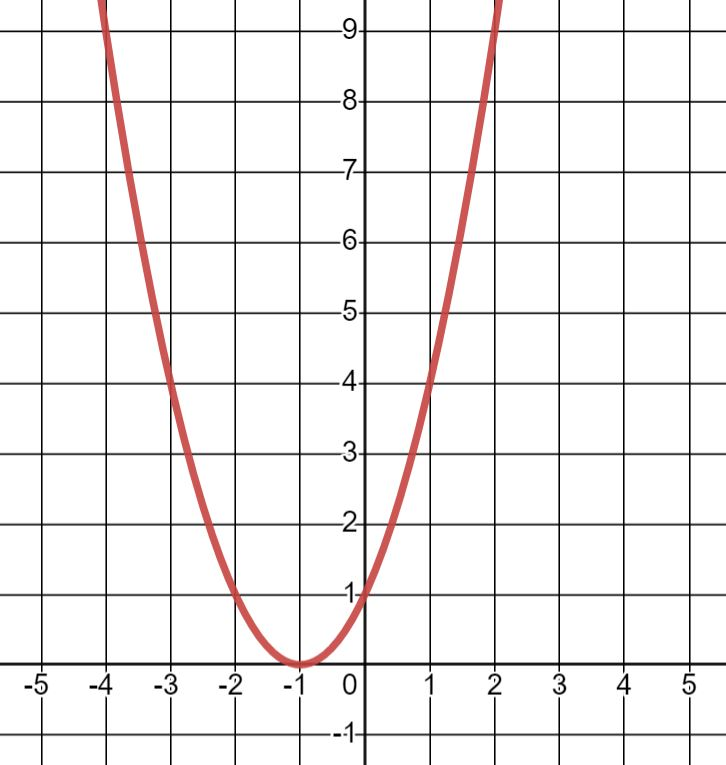###### Solution
1. For $$f(2)$$, our input or $$x$$ value is $$x = 2$$. Therefore, to compute this using the graph, starting at the origin (0, 0), we go two units to the right and then go up until we hit the graph. The corresponding $$y$$ value is 9. So $$f(2) = 9$$.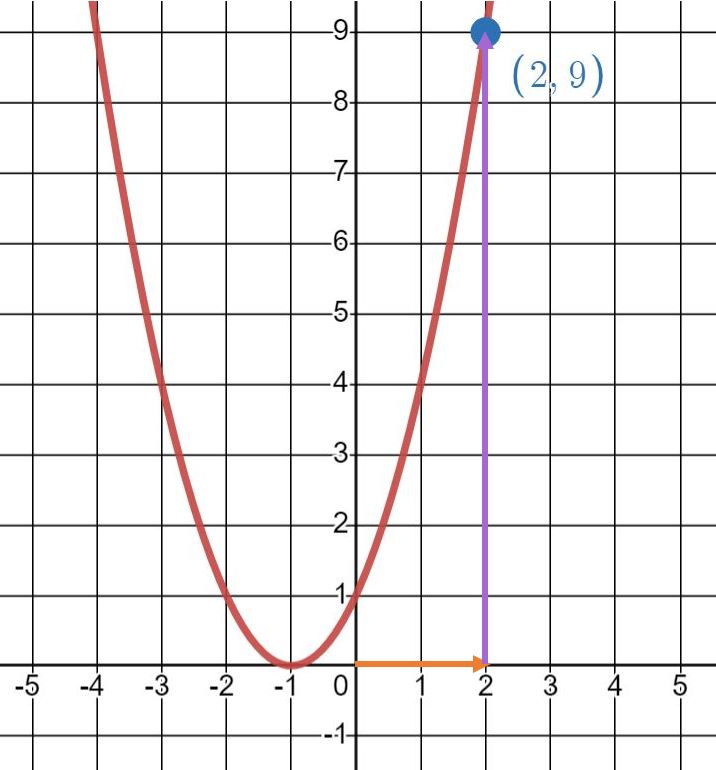We can compare this answer to what we get by plugging 2 into $$f$$. We have $$f(2) = (2 + 1)^2 = 3^2 = 9$$; this agrees with the answer from the graph!

1. For $$f(-3)$$, the input is $$x = -3$$. So using the graph, we move 3 units to the left then go up until we hit the graph. The $$y$$ value we get is 4. This means that $$f(-3) = 4$$.If we want to check our answer by just evaluating $$f(-3)$$ by plugging in -3 for $$x$$, we have $$f(-3) = (-3 + 1)^2 = (-2)^2 = 4$$.

1. Finally, for $$f(-1)$$, we move left 1 unit. Since the $$y$$ value for $$x = -1$$ is 0, we have $$f(-1) = 0$$.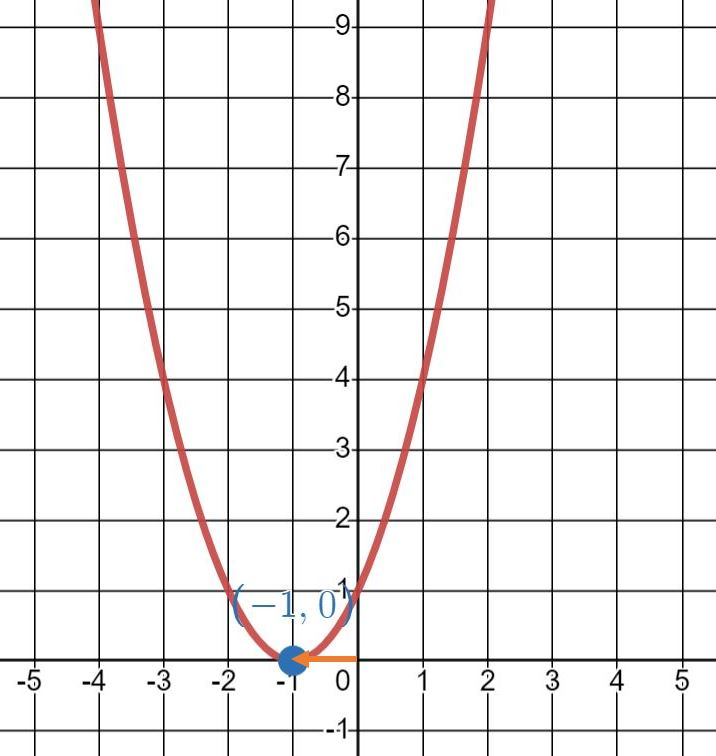We can plug in -1; we get $$f(-1) = (-1 + 1)^2 = 0^2 = 0$$.

##### Exercise $$\PageIndex{2}$$

Use the graph below to determine the following values for $$f(x) = |x - 2| - 3$$:

1. $$f(0)$$
2. $$f(-3)$$
3. $$f(4)$$

After determining these values, compare your answers to what you would get by simply plugging the given values into the function.1. For $$f(0)$$, the input is $$x = 0$$. Therefore, we just start at 0 and do not need to move horizontally. Since the graph is below the $$x$$-axis, we move down until we hit the graph. The graph has $$y = -1$$ there. Therefore, $$f(0) = -1$$.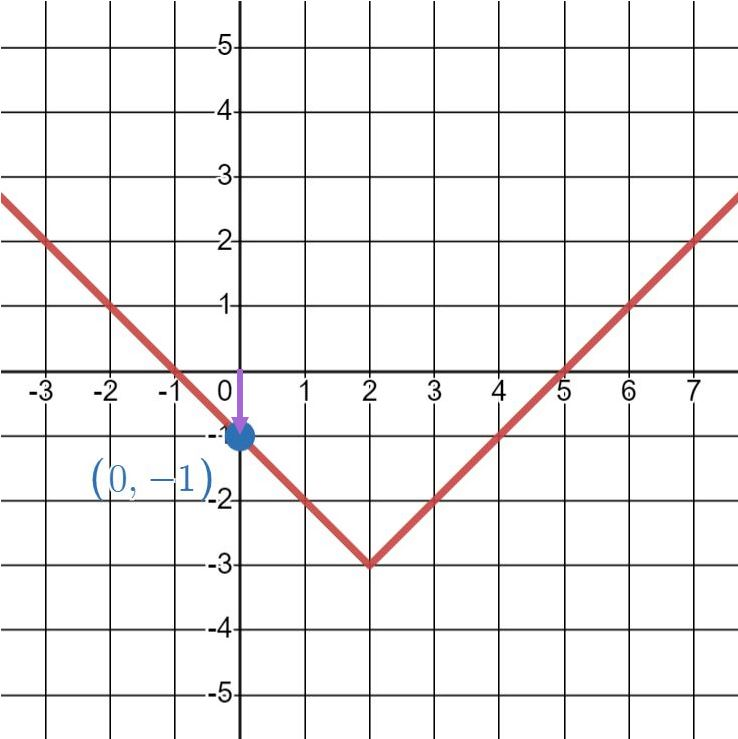We can also just evaluate the function directly. Plugging in $$x = 0$$, we get $$f(0) = |0 - 2| - 3 = |-2| - 3 = 2 - 3 = -1$$.

1. For $$f(-3)$$, we have the input $$x = -3$$. To find the output, we move left 3 units and then up until we hit the graph. There $$y = 2$$, so $$f(-3) = 2).We can plug in \(x = -3$$ to get $$f(-3) = |-3 - 2| - 3 = |-5| - 3 = 5 - 3 = 2$$.

1. Last, we need $$f(4)$$; this means $$x = 4$$. We move 4 units to the right, then 1 unit down. The $$y$$ value is $$y = -1$$. Therefore, $$f(4) = -1$$.We can compare this answer to what we get by plugging in $$x = 4$$. We have $$f(4) = |4 - 2| - 3 = |2| - 3 = 2 - 3 = -1$$.

## Determining if a Graph Represents a Function

The examples above were graphs of functions, but in the last section we talked about graphing relations and not just functions. However, functions are going to be the focus of what we work with in this course so this brings us to an important question: how do we know if a graph represents a function?

The big idea here lies in the definition of a function: each input (or $$x$$ value) may have only one output (or $$y$$ value). Based on this, we use what's called the vertical line test to determine if a graph represents a function or not. This test helps us identify from the graph of a function if there's anywhere that a single input may have two outputs.

##### Definition: VERTICAL LINE TEST

If a vertical line drawn anywhere on the graph of a relation only intersects the graph at one point, then that graph represents a function. If a vertical line can intersect the graph at two or more points, then the graph does not represent a function.

In other words, if a vertical line drawn anywhere only intersects the graph at only one spot, this means that each $$x$$ value corresponds to only one $$y$$ value, so the graph represents a function. If a vertical line can be drawn that intersects the graph at more than one spot, then this means that there is an $$x$$ value that corresponds to multiple $$y$$ values so the graph cannot represent a function.

Let's try this out on some examples!

##### Example $$\PageIndex{3}$$

Use the vertical line test to determine if the following graphs represent functions.###### Solution
1. Anywhere we draw a vertical line on this graph, it will only intersect the graph once. So the first graph represents a function!1. Anywhere we draw a vertical line on this graph, it will only intersect the graph once. So the second graph represents a function as well!1. Since we can draw a vertical line that intersects the graph at two places, this graph does not represent a function!##### Exercise $$\PageIndex{4}$$

Use the vertical line test to determine if the following graphs represent a function: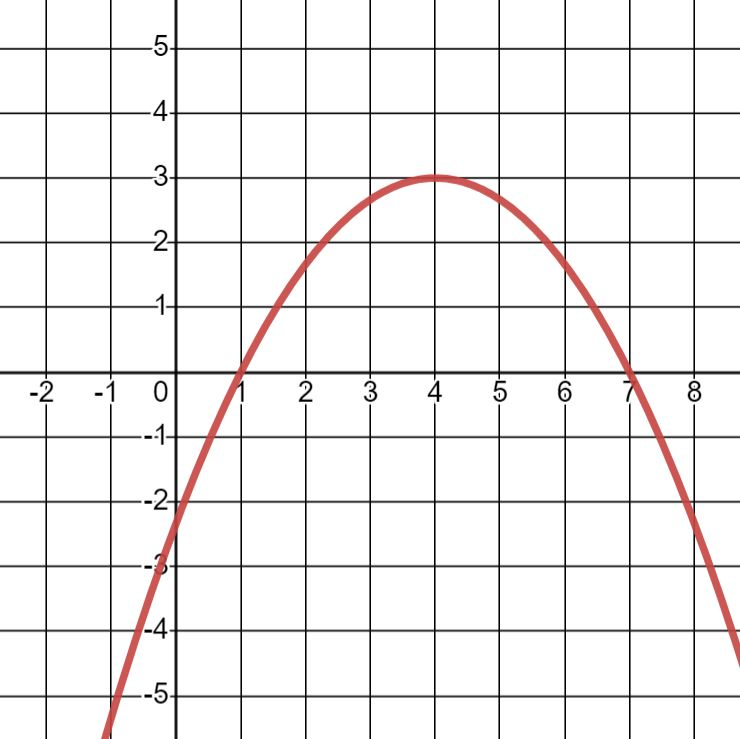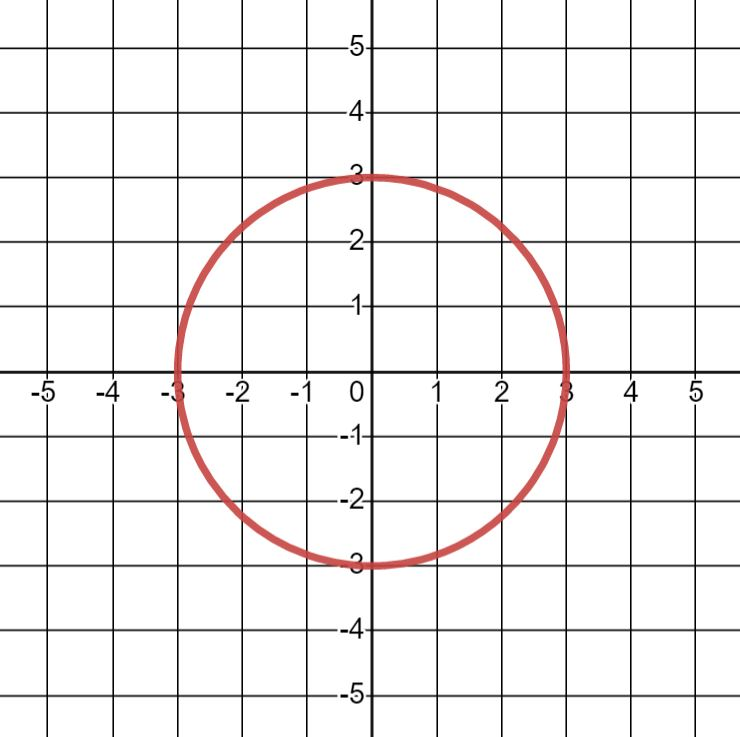1. Anywhere we draw a vertical line on this graph, it will only intersect the graph once. So the first graph represents a function!1. Since we can draw a vertical line that intersects the graph at two places, this graph does not represent a function!1. This graph is a little strange. Most places we draw a vertical line, it's easy to see that the line only intersects the graph at one point. Drawing a line at $$x = 0$$, it's not quite so clear. However, the graph does not appear to ever exist there, so there is nowhere for a vertical line to intersect. Therefore, the graph passes the vertical line test and represents a function!## Domain and Range of a Function on a Graph

We conclude this section by looking at how domain and range appear on a graph. First, let's look at definitions for the domain and range of a function that will be more helpful to us here. These definitions are the same as the ones that we used before, just restated for this context:

##### Definition: DOMAIN

The domain of a function is the collection of all possible inputs (or $$x$$ values) for the function.

##### Definition: RANGE

The range of a function is the collection of all possible outputs (or $$y$$ values) for the function.

This means that to determine the domain and range of a function using a graph, we just need to look at what is happening along the $$x$$ and $$y$$ axes. The values along the $$x$$ axis that are included on the graph create the domain of the function, while the values along the $$y$$ axis that are included on the graph create the range of the function. Let's look at some examples.

##### Example $$\PageIndex{5}$$

For each graph below, determine the domain and range of the function it represents.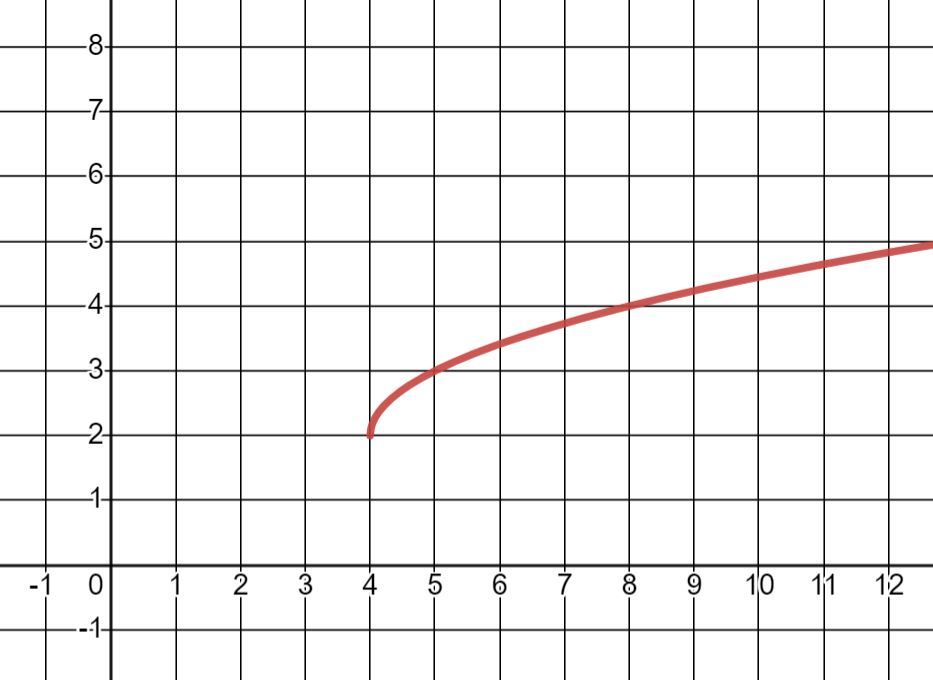###### Solution
1. To determine the domain, we look at all the possible $$x$$ values that the graph will reach. Here, the graph will continue to stretch across all the $$x$$ values, so the domain is all real numbers, that is, $$D = (-\infty, \infty)$$.To determine the range, we look at all the possible $$y$$ values that the graph can reach. The graph will continue growing both upwards and downwards without end, so the range is all real numbers, that is, $$R = (-\infty, \infty)$$.1. The second graph will expand outwards in each direction horizontally without stopping, so it will eventually include every possible $$x$$ value. Therefore, the domain is $$D = (-\infty, \infty)$$.Looking in the vertical direction to determine the range, the graph starts at $$y = 0$$ and grows upward toward infinity, so it will hit all $$y$$ values that are greater than or equal to 0. Therefore, the range will be $$y \geq 0$$.1. Finally, for the domain of the third function, looking at the horizontal direction, the graph starts at $$x = 4$$ and goes to the right toward infinity. Therefore, the possible inputs are any $$x$$ value that is greater than or equal to 4. In other words, the domain is $$x \geq 4$$.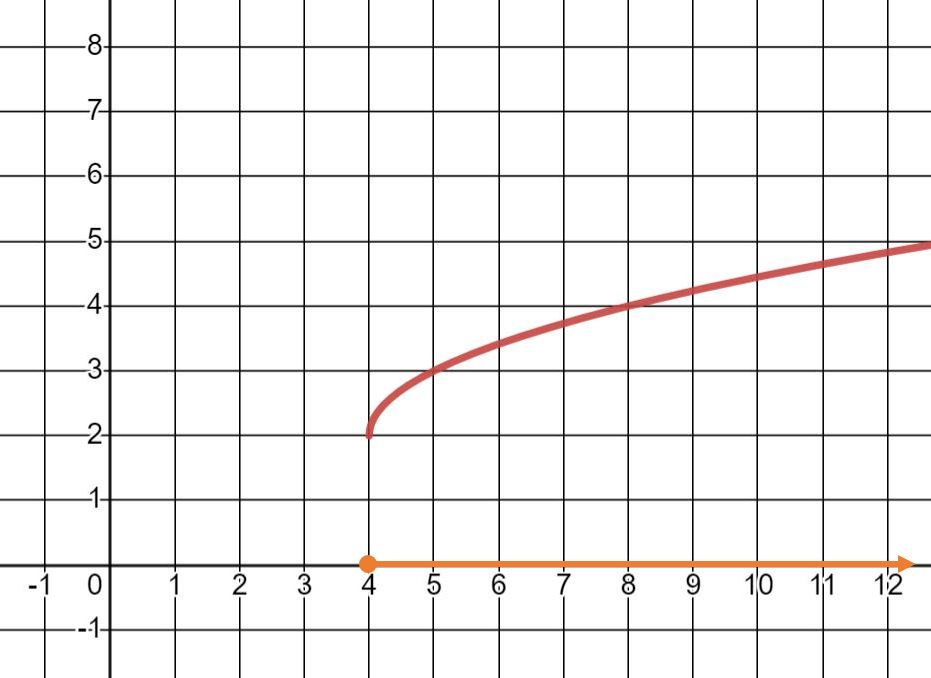For the range, we look in the vertical direction. The $$y$$ values start at $$y = 2$$, and even though the graph appears to be more horizontal than vertical, there is no limit on how much it is going to grow (even though it looks like it's slowing down!). Therefore, the possible output values are the $$y$$ values that are greater than or equal to 2. Therefore, the range is $$y \geq 2$$.##### Exercise $$\PageIndex{6}$$

For each graph below, determine the domain and range of the function it represents.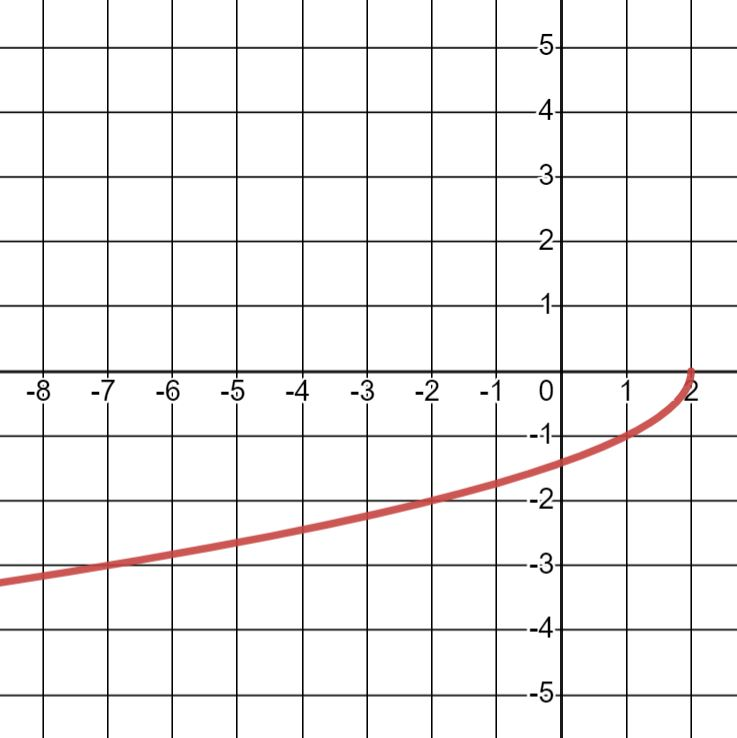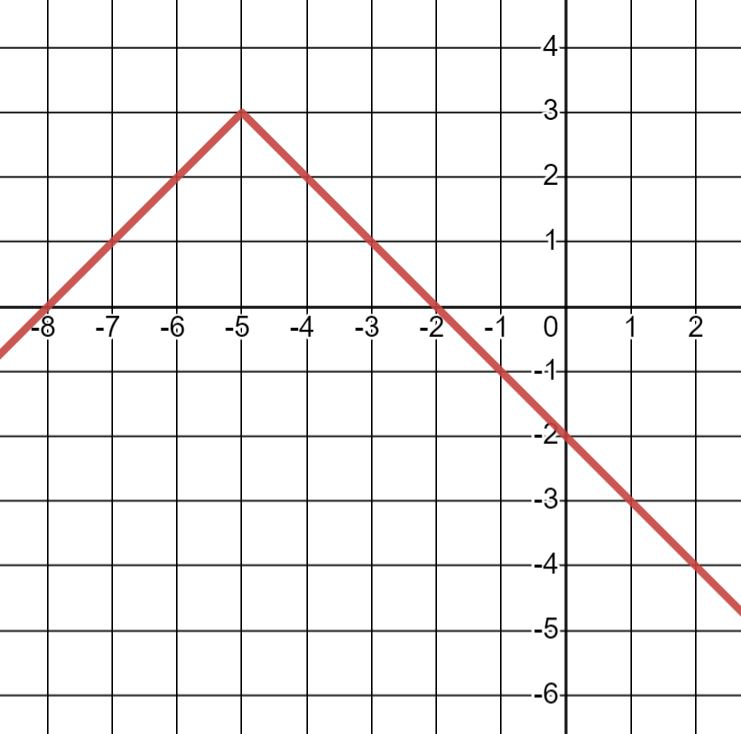Add texts here. Do not delete this text first.

1. To determine the domain, we look at all the possible $$x$$ values that the graph will reach. Here, the graph will continue to stretch across all the $$x$$ values, so the domain is all real numbers, that is, $$D = (-\infty, \infty)$$.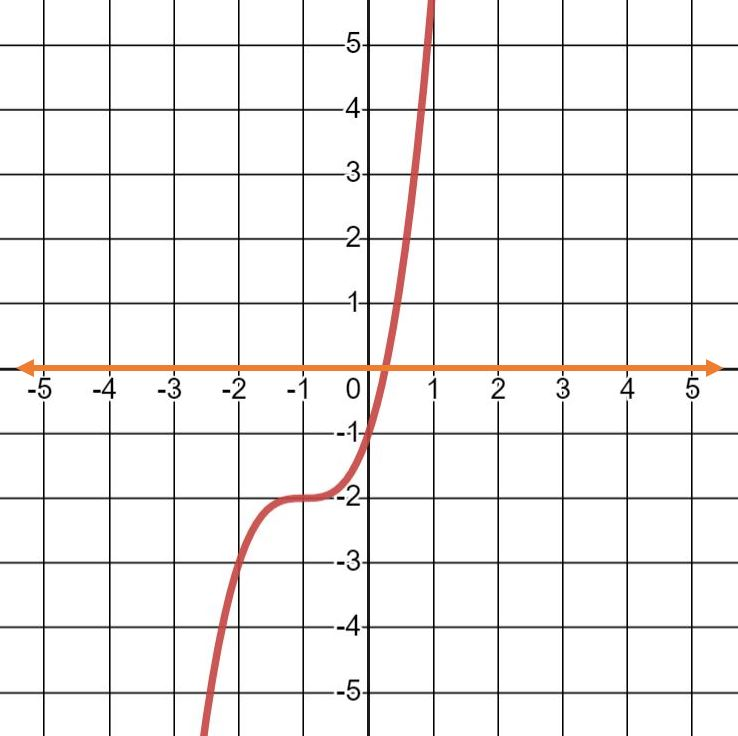To determine the range, we look at all the possible $$y$$ values that the graph can reach. The graph will continue growing both upwards and downwards without end, so the range is all real numbers, that is, $$R = (-\infty, \infty)$$.1. To determine the domain, looking in the horizontal direction, we see that the graph begins at $$x = 2$$ and extends to the left toward infinity. This means that our domain will include all numbers less than or equal to $$x = 2$$. Therefore, the domain is $$x \leq 2$$.For the range, we look in the vertical direction. This graph starts at $$y = 0$$ and goes downward. Similar to the graph in the last example, it appears to be more horizontal than vertical. However, it will continue downward without stopping, so the range will include all the negative $$y$$ values and 0. That is, the range is $$y \leq 0$$.1. For the last graph, to get the domain, we notice that the graph continuously extends horizontally and will eventually hit every possible value of $$x$$. Therefore, the domain will include all real numbers so $$D = (-\infty, \infty)$$.For the range, the $$y$$ values begin at $$y = 3$$ and then continue downward without stopping. The range is therefore any $$y$$ values that are less than or equal to 3. In other words, the range is $$y \leq 3$$.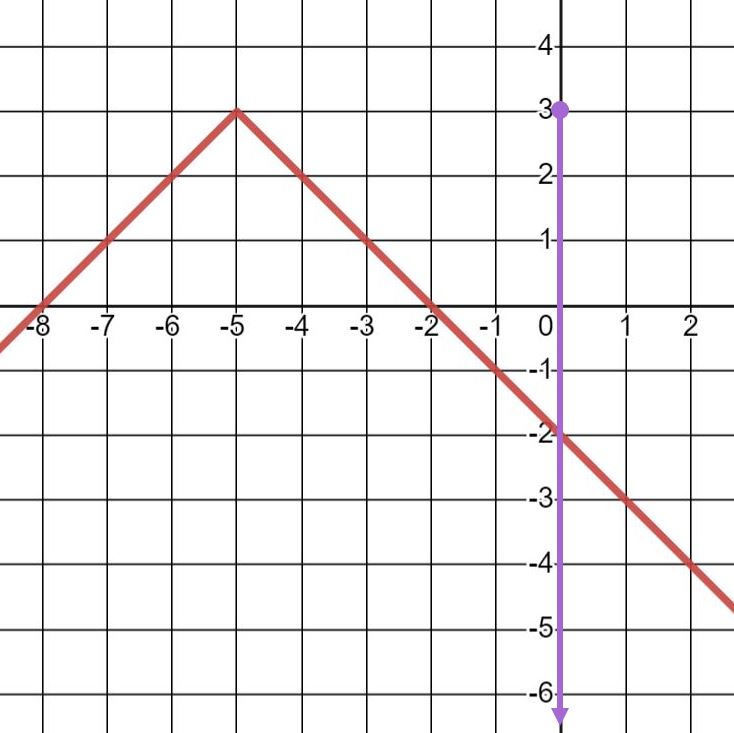## Key Concepts

• How to determine the value of a function $$f(x)$$ using a graph
1. Go to the point on the $$x$$ axis corresponding to the input for the function.
2. Move up or down until you hit the graph.
3. The $$y$$ value at that point on the graph is the value for $$f(x)$$.
• How to use the vertical line test to determine if a graph represents a function
1. Look for places where a vertical line can be drawn on the graph that might hit the graph in more than one place.
1. If it is possible to draw a vertical line that hits the graph in two or more places, the graph does NOT represent a function.
2. If any vertical line drawn hits the graph in only one place, the graph does represent a function.
• How to determine domain and range of a function using a graph
1. To determine the domain, look at the values along the $$x$$ axis that the graph reaches.
2. To determine the range, look at the values along the $$y$$ axis that the graph reaches.

This page titled 2.3: Understanding Graphs of Functions is shared under a CC BY-NC-SA 2.5 license and was authored, remixed, and/or curated by David Arnold.